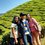# A Projectile Proof

1) A boy throws a stone to a building a horizontal distance x away through a window of height h above the launching point. The initial (launching) speed is v. "a" is the angle of the initial velocity with the horizontal. Using the projectile motion equations, prove that:

(tan a)^{2} - (2v^{2} / gx) (tan a) + [1 + (2 v^{2} h / g x^{2}) ] = 0, where g=9.8 m/s^{2}.

2) By solving for tan a in the above equation, find a condition for h such that there will be only 1 angle which will allow the stone to hit the window.Note by Panshul Rastogi
6 years, 2 months ago

This discussion board is a place to discuss our Daily Challenges and the math and science related to those challenges. Explanations are more than just a solution — they should explain the steps and thinking strategies that you used to obtain the solution. Comments should further the discussion of math and science.

When posting on Brilliant:

• Use the emojis to react to an explanation, whether you're congratulating a job well done , or just really confused .
• Ask specific questions about the challenge or the steps in somebody's explanation. Well-posed questions can add a lot to the discussion, but posting "I don't understand!" doesn't help anyone.
• Try to contribute something new to the discussion, whether it is an extension, generalization or other idea related to the challenge.
• Stay on topic — we're all here to learn more about math and science, not to hear about your favorite get-rich-quick scheme or current world events.

MarkdownAppears as
*italics* or _italics_ italics
**bold** or __bold__ bold
- bulleted- list
• bulleted
• list
1. numbered2. list
1. numbered
2. list
Note: you must add a full line of space before and after lists for them to show up correctly
paragraph 1paragraph 2

paragraph 1

paragraph 2

[example link](https://brilliant.org)example link
> This is a quote
This is a quote
    # I indented these lines
# 4 spaces, and now they show
# up as a code block.

print "hello world"
# I indented these lines
# 4 spaces, and now they show
# up as a code block.

print "hello world"
MathAppears as
Remember to wrap math in $$ ... $$ or $ ... $ to ensure proper formatting.
2 \times 3 $2 \times 3$
2^{34} $2^{34}$
a_{i-1} $a_{i-1}$
\frac{2}{3} $\frac{2}{3}$
\sqrt{2} $\sqrt{2}$
\sum_{i=1}^3 $\sum_{i=1}^3$
\sin \theta $\sin \theta$
\boxed{123} $\boxed{123}$

## Comments

Sort by:

Top Newest

i m now posting the proof of this....

Start with the basic setup:

Angle of velocity to horizontal = a Split velocity into Vx and Vy (velocity along x and y lines respectively). From basic pythag - V^2 = Vx^2 + Vy^2 Also, Vy/Vx = tan(a)

Projectile equations: x = Vx t y = Vy t - 1/2 g t^2

So h = Vy T - 1/2 g T^2 where T = time to reach height h

At this same time, x = Vx T

So T = x / Vx = x tan(a) / Vy

Substitute that into the equation for h: h = Vy x tan(a) / Vy - 1/2 g (x tan(a) / Vy)^2

We can simplify to: h = x tan(a) - 1/2 g (x tan(a) / Vy)^2

Now we also know that Vy = V sin(a)

So: h = x tan(a) - 1/2 g x^2 tan(a)^2 / (V^2 sin(a)^2)

tan(a)^2 / sin(a)^2 = 1/cos(a)^2 = sec(a)^2 sec(a)^2 = 1 - tan(a)^2

So, substituting all that in, we get:

h = x tan(a) - g x^2 / 2 V^2

This then reduces, eventually to the equation you're trying to prove.

For the second part... Simplify the expressions in the above equation to something like: Z^2 - pZ + (1+hp) = 0 where Z = tan(a) and p = 2 V^2 / gx^2

Solving for Z using the [-b +/- sqrt(b^2 - 4ac)]/2a will only give one result where b^2 - 4ac = 0

In this instance, b = p, a = 1 and c = (1+hp).

So b^2 - 4ac = p^2 - 4 - 4hp

Solve to zero... - is there any value of h which will allow that equation to equal zero? Remember that p is 2V^2/gx^2, so any condition of h that will satisfy this equation = 0 will most likely be related to V and x....

So... merely by rearranging it you get h = (p^2 - 4) / 4p

- 6 years, 2 months ago

Log in to reply

Where did you find this question ? Are you sure this is correct ?

- 6 years, 2 months ago

Log in to reply

yes..

- 6 years, 2 months ago

Log in to reply

Panshul please use latex code

- 6 years, 2 months ago

Log in to reply

wat is dat???

- 6 years, 2 months ago

Log in to reply

See this

- 6 years, 2 months ago

Log in to reply

${ x }^{ y }\frac { \sin { \left( a \right) } }{ x }$ this

- 6 years, 2 months ago

Log in to reply

$this$

- 6 years, 2 months ago

Log in to reply

i dont know how to use it... :(

- 6 years, 2 months ago

Log in to reply

Look up here. It's a very good note for beginners:)

- 6 years, 2 months ago

Log in to reply

×

Problem Loading...

Note Loading...

Set Loading...